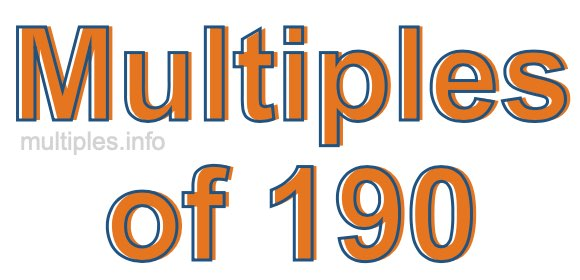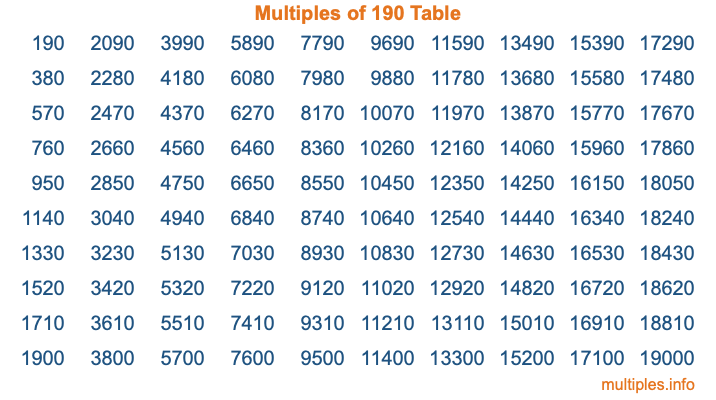Multiples of 190Welcome to the Multiples of 190 page. Here we will first teach you everything you will ever need to know about the multiples of 190, and then give you a study guide summary of everything we taught you to make sure you remember it all. Use this page to look up facts and learn information about the multiples of 190. This page will make you a multiples of one hundred ninety expert!

Definition of Multiples of 190
Multiples of 190 are all the numbers that when divided by 190 equal an integer. Each of the multiples of 190 are called a multiple. A multiple of 190 is created by multiplying 190 by an integer.

Therefore, to create a list of multiples of 190, you start with 1 multiplied by 190, then 2 multiplied by 190, then 3 multiplied by 190, and so on for as long as you want. Thus, the list of the first five multiples of 190 is 190, 380, 570, 760, and 950. To see a larger list of multiples of 190, see the printable image of Multiples of 190 further down on this page. We also have a category where you can choose any nth multiple of 190.

Multiples of 190 Checker
The Multiples of 190 Checker below checks to see if any number of your choice is a multiple of 190. In other words, it checks to see if there is any number (integer) that when multiplied by 190 will equal your number. To do that, we divide your number by 190. If the the quotient is an integer, then your number is a multiple of 190.

Is  a multiple of 190?

Least Common Multiple of 190 and ...
A Least Common Multiple (LCM) is the lowest multiple that two or more numbers have in common. This is also called the smallest common multiple or lowest common multiple and is useful to know when you are adding our subtracting fractions. Enter one or more numbers below (190 is already entered) to find the LCM.

Check out our LCM Calculator if you need more details about the Least Common Multiple or if you need the LCM for different numbers for adding and subtraction fractions.

nth Multiple of 190
As we stated above, 190 is the first multiple of 190, 380 is the second multiple of 190, 570 is the third multiple of 190, and so on. Enter a number below to find the nth multiple of 190.

th multiple of 190

Multiples of 190 vs Factors of 190
190 is a multiple of 190 and a factor of 190, but that is where the similarities end. All postive multiples of 190 are 190 or greater than 190. All positive factors of 190 are 190 or less than 190.

Below is the beginning list of multiples of 190 and the factors of 190 so you can compare:

Multiples of 190: 190, 380, 570, 760, 950, etc.

Factors of 190: 1, 2, 5, 10, 19, 38, 95, 190

As you can see, the multiples of 190 are all the numbers that you can divide by 190 to get a whole number. The factors of 190, on the other hand, are all the whole numbers that you can multiply by another whole number to get 190.

It's also interesting to note that if a number (x) is a factor of 190, then 190 will also be a multiple of that number (x).

Multiples of 190 vs Divisors of 190
The divisors of 190 are all the integers that 190 can be divided by evenly. Below is a list of the divisors of 190.

Divisors of 190: 1, 2, 5, 10, 19, 38, 95, 190

The interesting thing to note here is that if you take any multiple of 190 and divide it by a divisor of 190, you will see that the quotient is an integer.

Multiples of 190 Table
Below is an image of the first 100 multiples of 190 in a table. The table is in chronological order, column by column. The first column has the first ten multiples of 190, the second column has the next ten multiples of 190, and so on.The Multiples of 190 Table is also referred to as the 190 Times Table or Times Table of 190. You are welcome to print out our table for your studies.

Negative Multiples of 190
Although not often discussed or needed in math, it is worth mentioning that you can make a list of negative multiples of 190 by multiplying 190 by -1, then by -2, then by -3, and so on, to get the following list of negative multiples of 190:

-190, -380, -570, -760, -950, etc.

Multiples of 190 Summary
Below is a summary of important Multiples of 190 facts that we have discussed on this page. To retain the knowledge on this page, we recommend that you read through the summary and explain to yourself or a study partner why they hold true.

There are an infinite number of multiples of 190.

A multiple of 190 divided by 190 will equal a whole number.

190 divided by a factor of 190 equals a divisor of 190.

The nth multiple of 190 is n times 190.

The largest factor of 190 is equal to the first positive multiple of 190.

190 is a multiple of every factor of 190.

190 is a multiple of 190.

A multiple of 190 divided by a divisor of 190 equals an integer.

190 divided by a divisor of 190 equals a factor of 190.

Any integer times 190 will equal a multiple of 190.

Multiples of a Number
Here you can get the multiples of another number, all with the same attention to detail as we did for multiples of 190 on this page.

Multiples of
Multiples of 191
Did you find our page about multiples of one hundred ninety educational? Do you want more knowledge? Check out the multiples of the next number on our list!